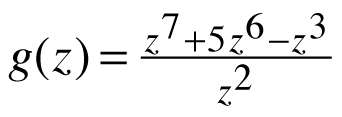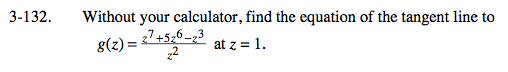### Home > CALC > Chapter 3 > Lesson 3.3.4 > Problem3-132

3-132.Without your calculator, find the equation of the tangent line toat x =1. Homework Help ✎Rewrite g(z) as a polynomial: g(z) = z5+5z4 = z

Find g '(z) by using the Power Rule.

Evaluate g '(z) at z = 1.